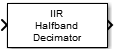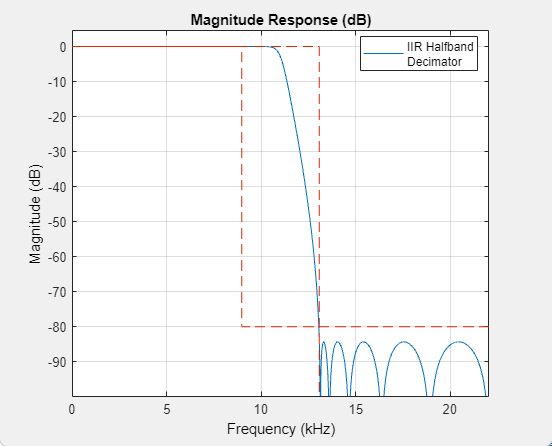# IIR Halfband Decimator

Decimate signal using polyphase IIR halfband filter

•Libraries:
DSP System Toolbox / Filtering / Multirate Filters

## Description

The IIR Halfband Decimator block performs polyphase decimation of the input signal by a factor of 2. To design the halfband filter, you can specify the block to use an elliptic design or a quasi-linear phase design. The block uses these design methods to compute the filter coefficients. To filter the inputs, the block uses a polyphase structure. The allpass filters in the polyphase structure are in a minimum multiplier form.

Elliptic design introduces nonlinear phase and creates the filter using fewer coefficients than the quasi-linear design. Quasi-linear phase design overcomes phase nonlinearity at the cost of additional coefficients.

Alternatively, instead of designing the halfband filter using a design method, you can specify the filter coefficients directly. When you choose this option, the allpass filters in the two branches of the polyphase implementation can be in a minimum multiplier form or a wave digital form.

You can also use the block to implement the analysis portion of a two-band filter bank to filter a signal into lowpass and highpass subbands. For more details, see Algorithms.

## Ports

### Input

expand all

Specify the input signal as a column vector or a matrix of size P-by-Q. If the input signal is a matrix, the block treats each column of the matrix as an independent channel. The number of rows in the input signal must be a multiple of 2.

This block supports variable-size input signals.

This port is unnamed until you select the Specify coefficients from input port parameter.

Data Types: `single` | `double`
Complex Number Support: Yes

Specify the allpass polynomial filter coefficients of the first branch as an N-by-`1` vector or N-by-`2` matrix of N first-order or second-order allpass sections.

#### Dependencies

To enable this parameter, set:

• Filter specification to `Coefficients`

• Internal allpass structure to `Minimum multiplier`

• Clear the Make the first branch a pure delay parameter

• Select the Specify coefficients from input port parameter

Data Types: `single` | `double` | `int8` | `int16` | `int32` | `int64` | `uint8` | `uint16` | `uint32` | `uint64` | `fixed point`

Specify the allpass polynomial filter coefficients of the second branch as an N-by-`1` vector or N-by-`2` matrix of N first-order or second-order allpass sections.

#### Dependencies

To enable this parameter, set:

• Filter specification to `Coefficients`

• Internal allpass structure to `Minimum multiplier`

• Select the Specify coefficients from input port parameter

Data Types: `single` | `double` | `int8` | `int16` | `int32` | `int64` | `uint8` | `uint16` | `uint32` | `uint64` | `fixed point`

### Output

expand all

Lowpass subband of the decimator output, returned as a column vector or a matrix of size P/2-by-Q. As the filter is a halfband filter, the downsampling factor is always 2.

This port is unnamed until you select the Output highpass subband parameter.

Data Types: `single` | `double`
Complex Number Support: Yes

Highpass subband of the decimator output, returned as a column vector or a matrix of size P/2-by-Q. As the filter is a halfband filter, the downsampling factor is always 2.

This port is unnamed until you select the Output highpass subband parameter.

Data Types: `single` | `double`
Complex Number Support: Yes

## Parameters

expand all

Select the parameters that the block uses to design the IIR halfband filter. Because the filter design has only two degrees of freedom, you can specify only two of the three parameters:

• ```Transition width and stopband attenuation``` (default) — Design the filter using Transition width (Hz) and Stopband attenuation (dB). This design is the minimum order design.

• `Filter order and transition width` — Design the filter using Filter order and Transition width (Hz).

• ```Filter order and stopband attenuation``` — Design the filter using Filter order and Stopband attenuation (dB).

• `Coefficients`— Specify the filter coefficients directly using the enabled parameters.

Specify the transition width of the IIR halfband filter as a real positive scalar in Hz. The transition width must be less than 1/2 the sample rate of the input signal.

#### Dependencies

To enable this parameter, set Filter specification to ```Filter order and transition width``` or ```Transition width and stopband attenuation```.

Specify the filter order as a finite positive integer. If you set Design method to `Elliptic`, then Filter order must be an odd integer greater than one. If you set Design method to `Quasi-linear phase`, then Filter order must be a multiple of four.

#### Dependencies

To enable this parameter, set Filter specification to `Filter order and transition width` or ```Filter order and stopband attenuation```.

Specify the minimum attenuation needed in the stopband of the IIR halfband filter as a real positive scalar in dB.

#### Dependencies

To enable this parameter, set Filter specification to ```Filter order and stopband attenuation``` or ```Transition width and stopband attenuation```.

Specify the design method for the IIR halfband filter.

• `Elliptic` — The filter has a nonlinear phase and uses few coefficients.

• `Quasi-linear phase` — The first branch of the polyphase filter structure is a pure delay, which results in an approximately linear phase response.

#### Dependencies

To enable this parameter, set Filter specification to any option except `Coefficients`.

Specify the internal allpass filter implementation structure as ```Minimum multiplier``` or ```Wave Digital Filter```. Each structure uses a different coefficients set, independently stored in the corresponding coefficients property.

#### Dependencies

To enable this parameter, set Filter specification to `Coefficients`.

When you select this check box, the first branch of the polyphase filter structure becomes a pure delay, and the Branch 1 allpass polynomial coefficients and Branch 1 Wave Digital coefficients parameters do not apply.

By default, this check box is not selected.

#### Dependencies

To enable this parameter, set Filter specification to `Coefficients`.

Specify the length of the first branch delay as a positive scalar.

#### Dependencies

To enable this parameter, set:

• Filter specification to `Coefficients`

• Select the Make the first branch a pure delay parameter

When you select this check box, you can input the branch 1 allpass polynomial coefficients and branch 2 allpass polynomial coefficients through the input ports coeffs1 and coeffs2. When you clear this check box, you specify the coefficients in the block dialog box through the Branch 1 allpass polynomial coefficients and Branch 2 allpass polynomial coefficients parameters.

#### Dependencies

To enable this parameter, set:

• Filter specification to `Coefficients`

• Internal allpass structure to `Minimum multiplier`

Specify the allpass polynomial filter coefficients of the first branch as an N-by-`1` vector or N-by-`2` matrix of N first-order or second-order allpass sections.

This parameter is tunable, that is, you can change its value during simulation.

Tunable: Yes

#### Dependencies

To enable this parameter, set:

• Filter specification to `Coefficients`

• Internal allpass structure to `Minimum multiplier`

• Clear the Make the first branch a pure delay parameter

• Clear the Specify coefficients from input port parameter

Specify the allpass polynomial filter coefficients of the second branch as an N-by-`1` vector or N-by-`2` matrix of N first-order or second-order allpass sections.

This parameter is tunable, that is, you can change its value during simulation.

Tunable: Yes

#### Dependencies

To enable this parameter, set:

• Filter specification to `Coefficients`

• Internal allpass structure to `Minimum multiplier`

• Clear the Specify coefficients from input port parameter

Specify the allpass filter coefficients of the first branch in wave digital filter (WDF) form as an N-by-`1` vector or N-by-`2` matrix of N first-order or second-order allpass sections.

The magnitude of each WDF coefficient must not be greater than 1.

#### Dependencies

To enable this parameter, set:

• Filter specification to `Coefficients`

• Internal allpass structure to `Wave Digital Filter`

• Clear the Make the first branch a pure delay parameter

Specify the allpass filter coefficients of the second branch in wave digital filter form as an N-by-`1` vector or N-by-`2` matrix of N first-order or second-order allpass sections.

The magnitude of each WDF coefficient must not be greater than 1.

#### Dependencies

To enable this parameter, set:

• Filter specification to `Coefficients`

• Internal allpass structure to `Wave Digital Filter`

When you select this check box, the block treats the last section of the second branch as a first order section. When the coefficients of the second branch are in an N-by-`2` matrix, the block ignores the second element of the last row of the matrix. The last section of the second branch then becomes a first-order section.

When you clear this check box, the block treats the last section of the second branch as a second-order section. When the coefficients of the second branch are in an N-by-`1` matrix, the block ignores this parameter.

#### Dependencies

To enable this parameter, set Filter specification to `Coefficients`.

When you select this check box, the block acts as an analysis filter bank, producing two power-complementary outputs. When you clear this check box, the block acts as an IIR halfband decimator and accepts a single vector or matrix as input.

When you select this check box, the block inherits its sample rate from the input signal. When you clear this check box, you specify the sample rate in Input sample rate (Hz).

Specify the sample rate of the input signal as a scalar in Hz.

#### Dependencies

To enable this parameter, clear the Inherit sample rate from input parameter.

Click this button to open the Filter Visualization Tool (FVTool) and display the magnitude and phase response of the IIR Halfband Decimator. The response is based on the values you specify in the block parameters dialog box. Changes made to these parameters update FVTool.To update the magnitude response while FVTool is running, modify the dialog box parameters and click .

Specify the type of simulation to run. You can set this parameter to:

• `Code generation` (default)

Simulate model using generated C code. The first time you run a simulation, Simulink® generates C code for the block. The C code is reused for subsequent simulations, as long as the model does not change. This option requires additional startup time but provides faster simulation speed than ```Interpreted execution```.

• `Interpreted execution`

Simulate model using the MATLAB®  interpreter. This option shortens startup time but has slower simulation speed than ```Code generation```.

## Block Characteristics

 Data Types `double` | `single` Direct Feedthrough `no` Multidimensional Signals `no` Variable-Size Signals `yes` Zero-Crossing Detection `no`

expand all

## Version History

Introduced in R2015b